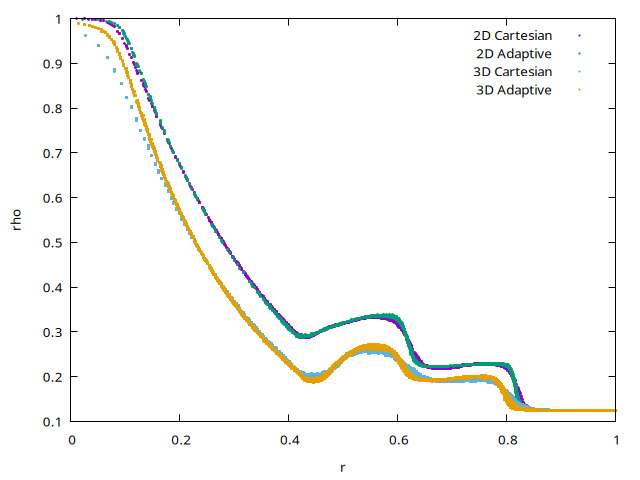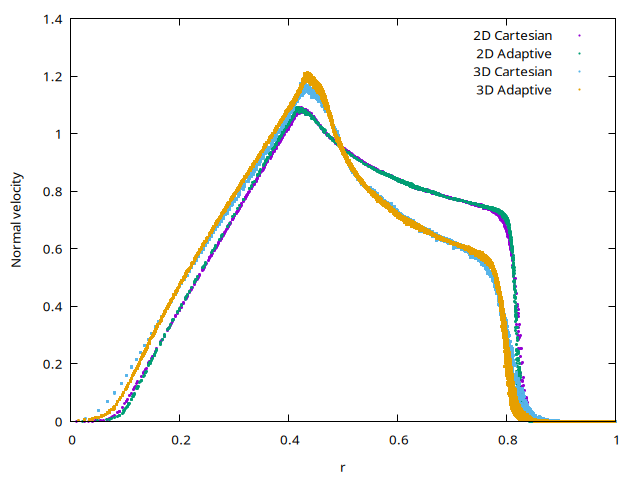# Two- and three-dimensional explosions

We solve the Euler equations for a compressible gas. We also need to compute volume fractions for the initial condition.

#include "compressible.h"
#include "fractions.h"

#if dimension == 2
# define LEVEL 7
#else // 3D
# define LEVEL 6
#endif

int main() {

We make boundary conditions free outflow.

  foreach_dimension() {
w.n[right] = neumann(0);
w.n[left]  = neumann(0);
}

The domain spans [-1:1]\times[-1:1]\times[-1:1].

  origin (-1, -1, -1);
size (2.);
init_grid (1 << LEVEL);
run();
}

Initial conditions come from Toro’s book (Riemann Solvers and Numerical Methods for Fluid Dynamics, 3rd Edition, Springer Ed.) Chapter 17 section 17.1.1 are given in terms of density (\rho), pression (p), velocity (u) both at the left and right side of the discontinuity placed at R=0.4.

event init (t = 0)
{
double R = 0.4 ;
double rhoL = 1., rhoR = 0.125 ;
double pL = 1.0,  pR = 0.1 ;

To define an accurate (i.e. symmetrical) initial sphere of rayon R, we compute the volume fraction corresponding to the spherical interface.

  scalar f[];
fraction (f, sq(x) + sq(y) + sq(z) - sq(R));

Left and right initial states for \rho, \mathbf{w} and energy E = \rho \mathbf{u}^2/2 + p/(\gamma-1).

  foreach() {
rho[] = rhoR*f[] + rhoL*(1. - f[]);
foreach_dimension()
w.x[] = 0.;
E[] = (pR*f[] + pL*(1. - f[]))/(gammao - 1.);
}

theta = 1.3; // tune limiting from the default minmod
}

event print (t = 0.25)
{

At t=0.25 we output the values of \rho and the normal velocity \mathbf{u}_n as functions of the radial coordinate.

  foreach() {
double r = sqrt(sq(x) + sq(y) + sq(z));
double wn = (w.x[]*x + w.y[]*y + w.z[]*z)/r;
printf ("%g %g %g\n", r, rho[], wn/rho[]);
}

For reference we also output a cross-section at y=0.

  for (double x = 0; x <= 1; x += 1e-2)
fprintf (stderr, "%g %.4f %.4f\n", x,
interpolate (rho, x, 0.),
interpolate (w.x, x, 0.));
}

On trees, we adapt the mesh by controlling the error on the density field.

#if TREE
adapt_wavelet ({rho}, (double[]){5e-3}, LEVEL + 1);
}
#endif

## Results

Results are presented in terms of \rho and normal velocity u_n for Cartesian (7 levels in 2D and 6 levels in 3D) and adaptive (8 levels in 2D and 7 levels in 3D) computations. The numerical results compare very well with Toro’s numerical experiments.

set xrange [0:1]
set xlabel 'r'

set term PNG enhanced font ",10"
set output 'rho.png'
set ylabel 'rho'
plot './cout' u 1:2 w p pt 7 ps 0.2 t '2D Cartesian', \
'./out' u 1:2 w p pt 7 ps 0.2 t '2D Adaptive', \
'../explosion3D/out' u 1:2 w p pt 7 ps 0.2 t '3D Cartesian', \
'../explosion.3D/out' u 1:2 w p pt 7 ps 0.2 t '3D Adaptive'set output 'velocity.png'
'../explosion.3D/out' u 1:3 w p pt 7 ps 0.2 t '3D Adaptive'